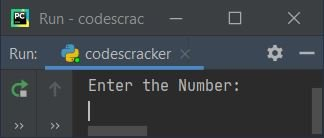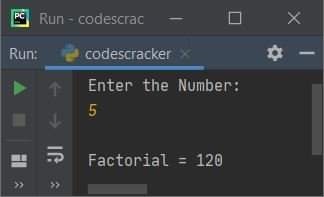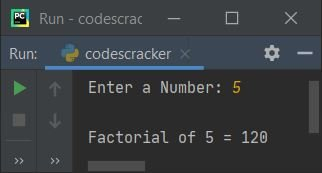# Python Program to Find Factorial of a Number

In this article, I've created some programs in Python, that find and prints factorial of a given number by user at run-time. Here are the list of approaches used:

• Find Factorial of a Number using while Loop
• Using for Loop
• Using user-defined Function
• Using Recursion
• Using Class

Before creating these programs, let's remind about factorial formula, that is:

`n! = (n)*(n-1)*(n-2)*...*3*2*1`

Here n indicates a number of which, the factorial is going to find. The ! (exclamation) indicates factorial. So n! can be called as n factorial. For example, 5! can be calculated as:

```5! = 5*4*3*2*1
= 120```

## Find Factorial using while Loop

To find factorial of any number in Python, you have to ask from user to enter the number, then find and print its factorial as per the formula given above, like shown in the program given below.

The question is, write a Python program to find factorial of a given number using while loop. Here is its answer:

```print("Enter the Number: ")
num = int(input())

fact = 1
i = 1
while i<=num:
fact = fact*i
i = i+1

print("\nFactorial =", fact)```

Here is the initial output produced by this Python program:Now supply the input say 5 as number and press `ENTER` key to find its factorial like shown in the snapshot given below:The dry run of above program with user input 5 goes like:

• Initial values, num=5 (entered by user), fact=1, i=1
• Now the execution of while loop begins
• That is, the condition i<=num or 1<=5 evaluates to be true, therefore program flow goes inside the loop (inside its body)
• Inside the loop, fact*i or 1*1 or 1 gets initialized to fact. And the value of i gets incremented by 1. So i=2
• Again the condition i<=num or 2<=5 evaluates to be true, therefore again fact*i or 1*2 or 2 gets initialized to fact. So fact=2
• And i+1 or 2+1 or 3 gets initialized to i. So i=3
• Again the condition, i<=num or 3<=5 evaluates to be true, therefore program flow again goes inside the loop and executes its two statements. This process continues until the condition evaluates to be false
• In this way, after exiting from the loop, the variable fact holds the factorial value of a number stored in num variable
• So just print the value of fact as factorial of a given number

## Find Factorial using for Loop

This program does the same job as of previous program, but using for loop, instead of while. The try-except is used in this program to handle with invalid inputs entered by user. And end is used to skip inserting an automatic newline.

```print("Enter a Number: ", end="")
try:
num = int(input())
fact = 1
for i in range(1, num+1):
fact = fact*i
print("\nFactorial of", num, "=", fact)
except ValueError:
print("\nInvalid Input!")```

Here is its sample run with same user input as of previous program:In above program, the following code:

`for i in range(1, num+1):`

is applied to execute the following statement:

`fact = fact*i`

num number of times with value of i from 1 to num's value.

## Find Factorial using Function

This program is created using a user-defined function named findFact(). This function receives a value as its argument, then find and returns its factorial.

```def findFact(n):
f = 1
for i in range(1, n+1):
f = f*i
return f

print("Enter a Number: ", end="")
try:
num = int(input())
fact = findFact(num)
print("\nFactorial of", num, "=", fact)
except ValueError:
print("\nInvalid Input!")```

This program produces same output as of previous program:

## Find Factorial using Recursion

The following program is created using a recursive function named findFact(). This function calls itself until the value of its argument becomes equal to 1.

```def findFact(n):
if n==1:
return n
else:
return n*findFact(n-1)

print("Enter a Number: ", end="")
try:
num = int(input())
fact = findFact(num)
print("\nFactorial of", num, "=", fact)
except ValueError:
print("\nInvalid Input!")```

## Find Factorial using Class

This is the last program to find and print factorial of a number entered by user, created using a class named CodesCracker. An object ob is created of this class to access its member function (findFact()) using dot (.) operator:

```class CodesCracker:
def findFact(self, n):
f = 1
for i in range(1, n + 1):
f = f * i
return f

print("Enter a Number: ", end="")
num = int(input())

ob = CodesCracker()
print("\nFactorial of", num, "=", ob.findFact(num))```

#### Same Program in Other Languages

Python Online Test

« Previous Program Next Program »